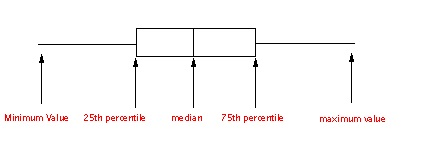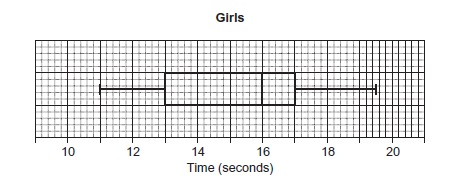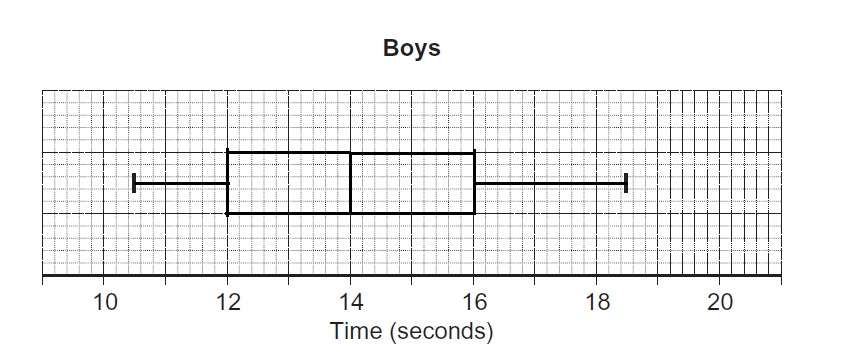# GCSE Maths: Boxplots## What do boxplots express?

Every boxplot gives a minimum, lower quartile, median, upper quartile and maximum as shown in the diagram below.## Remember

Range = Highest value – Lowest value

Interquartile Range = upper quartile – lower quartile

A small interquartile range shows the data is quite consistent while a large interquartile range shows that the data is quite inconsistent

Example

Girls and boys are timed in a race and the box plot gives information about the girls’ times.The following information is known about the boys’ times:

(1) 25% of the boys take 12 seconds or less

(2) The interquartile range for the boys is the same as for the girls

(3) The ratio of median times is girls : boys = 8:7

(4) The time of the fastest boy is 10.5 and the time of the slowest boy is 18.5.

Complete a box plot for the boys’ times.

Solutions

Lower quartile = 12 seconds

Interquartile range for the girls = 4 = Interquartile range for the boys

Upper quartile = 12 + 4 = 16

Boys’ median = 7/8 x girls’ median = 7/8 x 16 = 14

Fastest and slowest times are 10.5 and 18.5 seconds respectively### Work Towards That 'A' Grade

Achieve the results you want with-written revision resources when you register with iRevise.
Its Free!